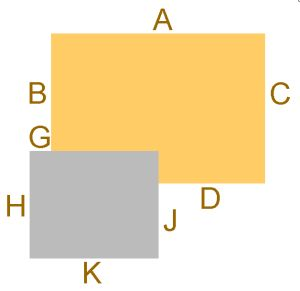01603 721590
Information
Search
UK
Set Delivery
Postcode
IP4
Empty
Setting the first half of your postcode shows you the full choice in your area.

Base dimensions

4.5m deep cabins (14'9")We recommend that your base should be no smaller than the floor size of the new log cabin and no more than a few inches larger.

Cabin size - 14'9" x 13'1" (4.5m x 4.0m)
Dimension A = 4000mm
Dimension B = 3500mm
Dimension C = 4500mm
Dimension D = 2000mm
Dimension G = 1000mm
Dimension H = 3000mm
Dimension J = 2000mm
Dimension K = 3000mm

Overall width from side to side (A) = 4000mm (excluding verandah)
Overall depth from front to back (C) = 4500mm (excluding verandah)

Cabin size - 14'9" x 14'9" (4.5m x 4.5m)
Dimension A = 4500mm
Dimension B = 3500mm
Dimension C = 4500mm
Dimension D = 2500mm
Dimension G = 1000mm
Dimension H = 3000mm
Dimension J = 2000mm
Dimension K = 3000mm

Overall width from side to side (A) = 4500mm (excluding verandah)
Overall depth from front to back (C) = 4500mm (excluding verandah)

Cabin size - 14'9" x 16'5" (4.5m x 5.0m)
Dimension A = 5000mm
Dimension B = 3500mm
Dimension C = 4500mm
Dimension D = 2500mm
Dimension G = 500mm
Dimension H = 3000mm
Dimension J = 2000mm
Dimension K = 3000mm

Overall width from side to side (A) = 5000mm (excluding verandah)
Overall depth from front to back (C) = 4500mm (excluding verandah)

Cabin size - 14'9" x 18'1" (4.5m x 5.5m)
Dimension A = 5500mm
Dimension B = 3500mm
Dimension C = 4500mm
Dimension D = 3000mm
Dimension G = 500mm
Dimension H = 3000mm
Dimension J = 2000mm
Dimension K = 3000mm

Overall width from side to side (A) = 5500mm (excluding verandah)
Overall depth from front to back (C) = 4500mm (excluding verandah)

Cabin size - 14'9" x 19'8" (4.5m x 6.0m)
Dimension A = 6000mm
Dimension B = 3500mm
Dimension C = 4500mm
Dimension D = 3500mm
Dimension G = 500mm
Dimension H = 3000mm
Dimension J = 2000mm
Dimension K = 3000mm

Overall width from side to side (A) = 6000mm (excluding verandah)
Overall depth from front to back (C) = 4500mm (excluding verandah)

CLEARANCE SALE . . . many prices reduced . . . see product pages for details!